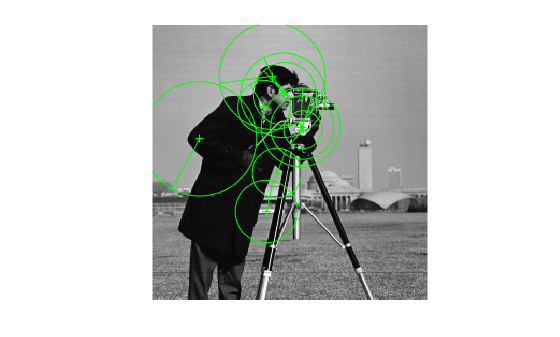# length

Number of stored points

## Syntax

``length(points)``

## Description

example

````length(points)` returns the number of stored points in the points object.```

## Examples

collapse all

`I = imread("cameraman.tif");`

Detect KAZE points from the image.

`points = detectKAZEFeatures(I);`

Find number of stored points.

`numPoints = length(points)`
```numPoints = 631 ```

Extract KAZE features from the detected points.

`[features,validPoints] = extractFeatures(I,points);`

Plot the ten strongest valid extracted points and show their orientations.

```imshow(I) hold on strongestPoints = selectStrongest(validPoints,10); plot(strongestPoints,showOrientation=true) hold off```## Input Arguments

collapse all

Points object,specified as one of the point feature objects described in Point Feature Types. The object contains information about the feature points detected in the input image. To obtain points, use the appropriate detect function that pairs with the point feature type.

## Version History

Introduced in R2017b